# Prime Factorization Unit 1 SWBAT Generate a model

• Slides: 19
Download presentationPrime Factorization Unit 1SWBAT Generate a model of prime factors and prime numbers n n Homework: Prime Factorization WS Do Now: Use a factor house to find the factors of 72 72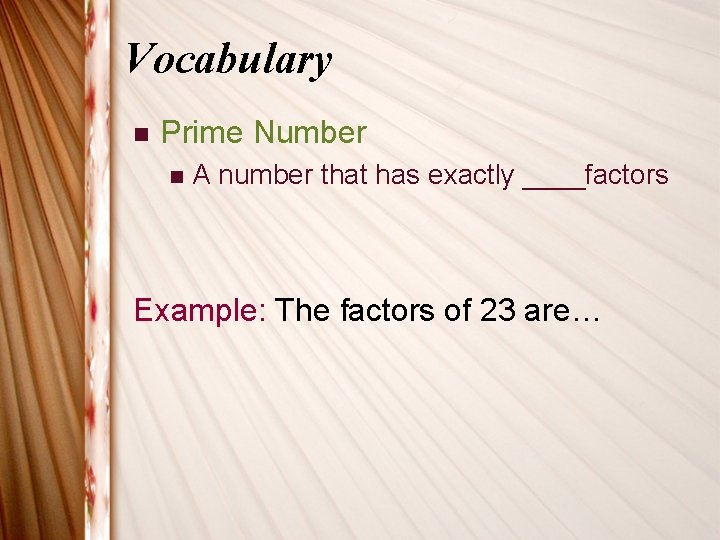Vocabulary n Prime Number n A number that has exactly ____factors Example: The factors of 23 are…Prime Factorization n To write a number as the _____of its _____factors n Circle the Prime Numbers: 2, 3, 4, 5, 6, 7, 8, 9, 10, 11, 12 , 13 , 14, 15, 16, 17, 18, 19, 20 21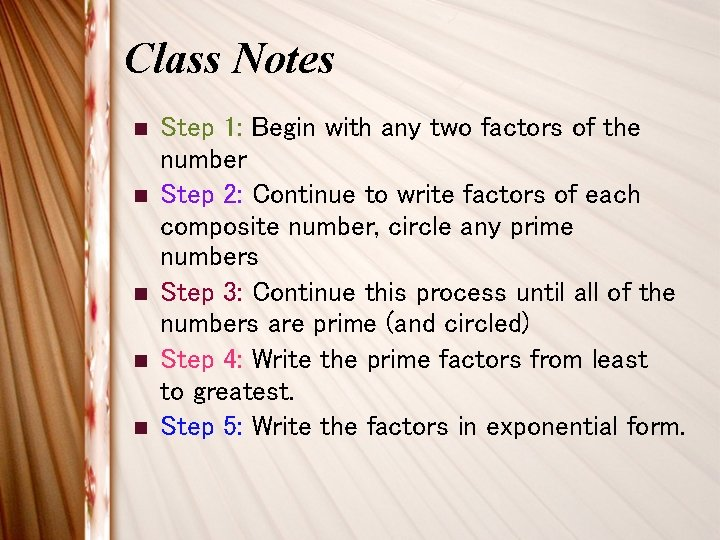Class Notes n n n Step 1: Begin with any two factors of the number Step 2: Continue to write factors of each composite number, circle any prime numbers Step 3: Continue this process until all of the numbers are prime (and circled) Step 4: Write the prime factors from least to greatest. Step 5: Write the factors in exponential form.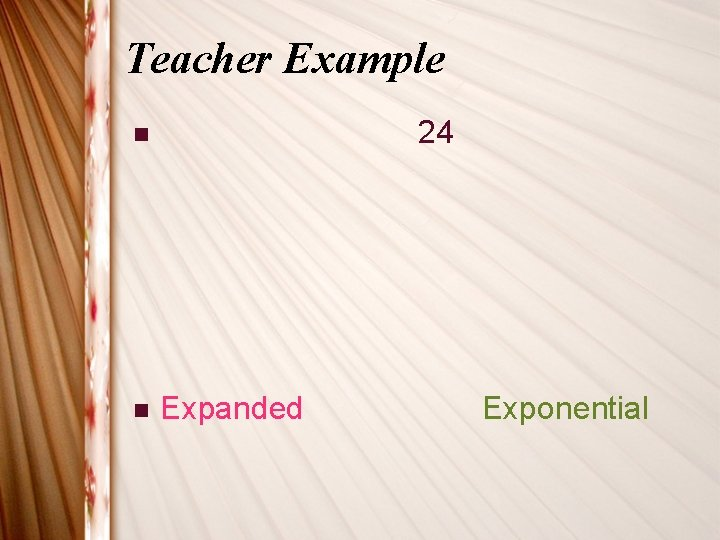Teacher Example 24 n n Expanded ExponentialScholar Example 32 n n Expanded Exponential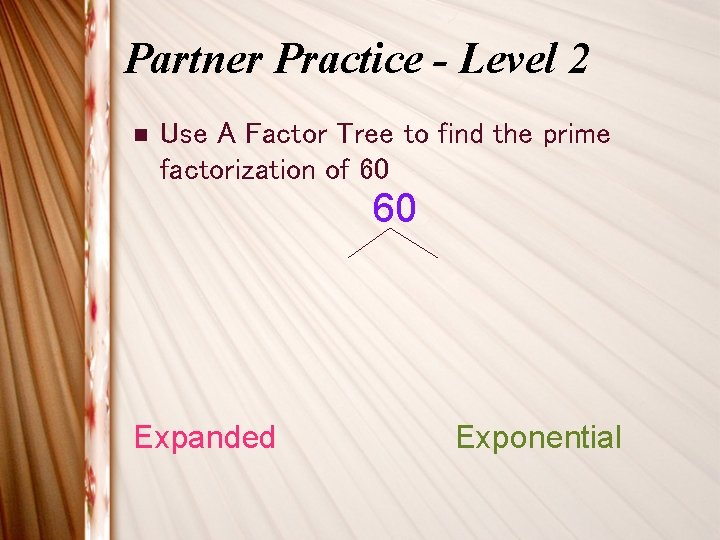Partner Practice - Level 2 n Use A Factor Tree to find the prime factorization of 60 60 Expanded Exponential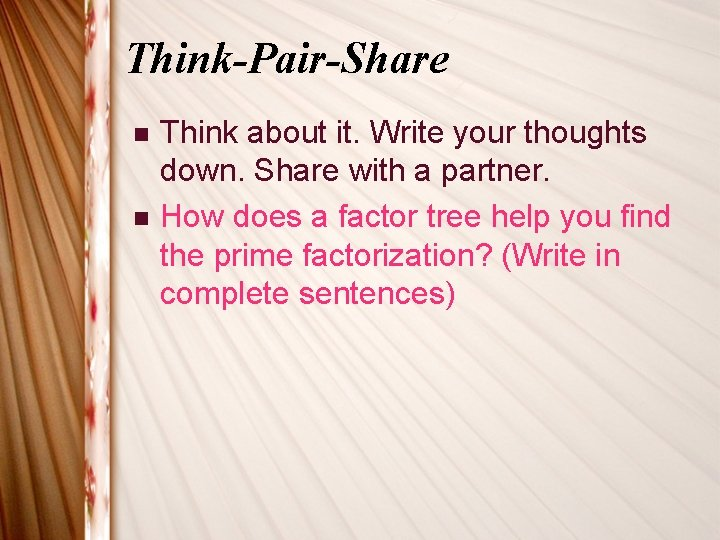Think-Pair-Share n n Think about it. Write your thoughts down. Share with a partner. How does a factor tree help you find the prime factorization? (Write in complete sentences)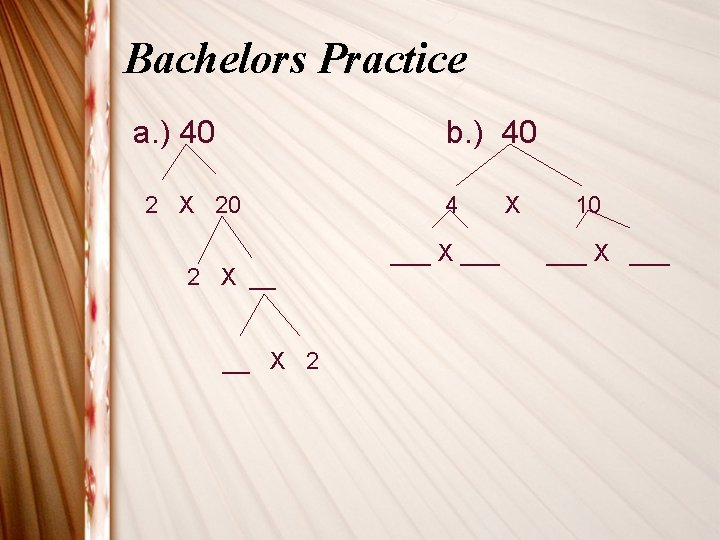Bachelors Practice a. ) 40 b. ) 40 2 X 20 2 X __ __ X 2 4 ___ X 10 ___ X ___Bachelors Practice 1 c 40 n 5 X 8 __ X __ ___ X ___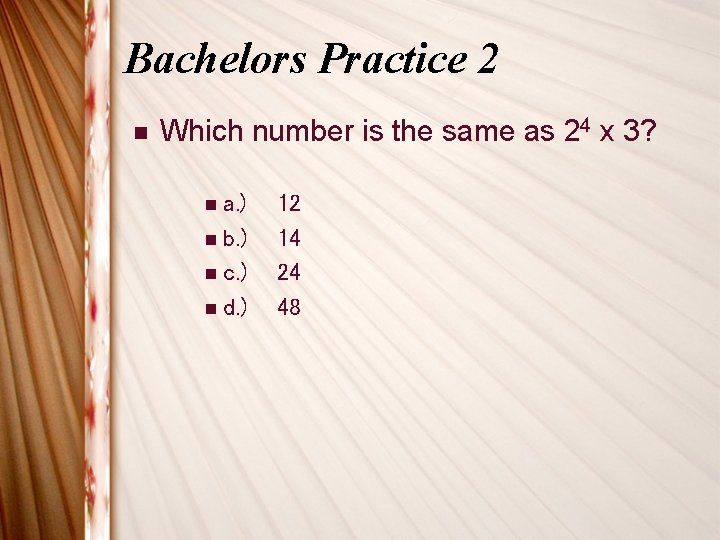Bachelors Practice 2 n Which number is the same as 24 x 3? n a. ) n b. ) n c. ) n d. ) 12 14 24 48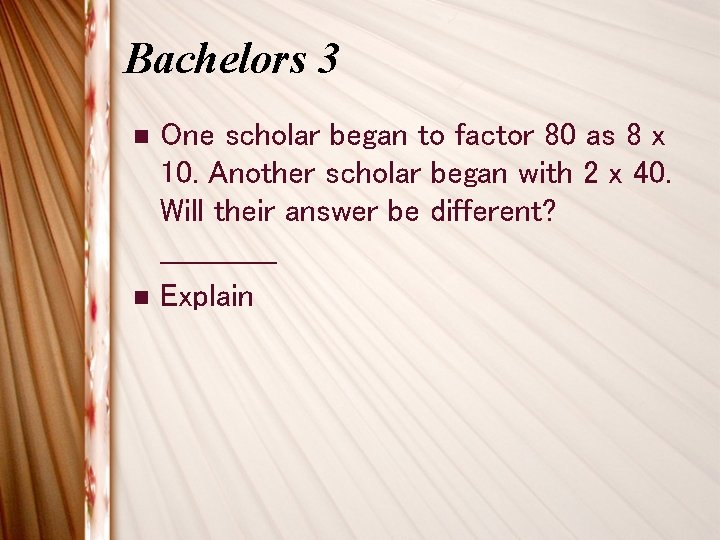Bachelors 3 n n One scholar began to factor 80 as 8 x 10. Another scholar began with 2 x 40. Will their answer be different? ______ Explain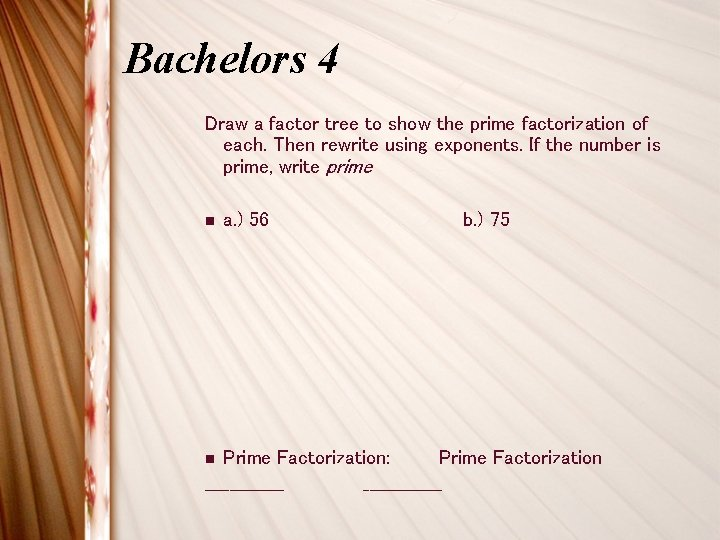Bachelors 4 Draw a factor tree to show the prime factorization of each. Then rewrite using exponents. If the number is prime, write prime n a. ) 56 b. ) 75 Prime Factorization: Prime Factorization _____________ n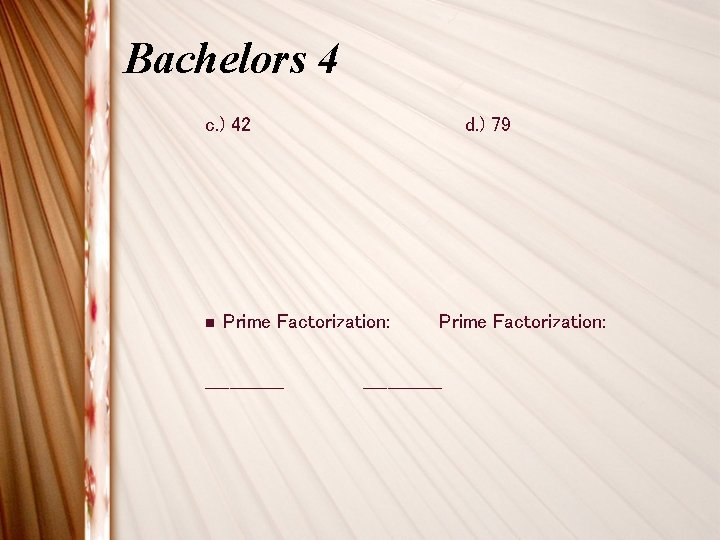Bachelors 4 c. ) 42 n d. ) 79 Prime Factorization: _____________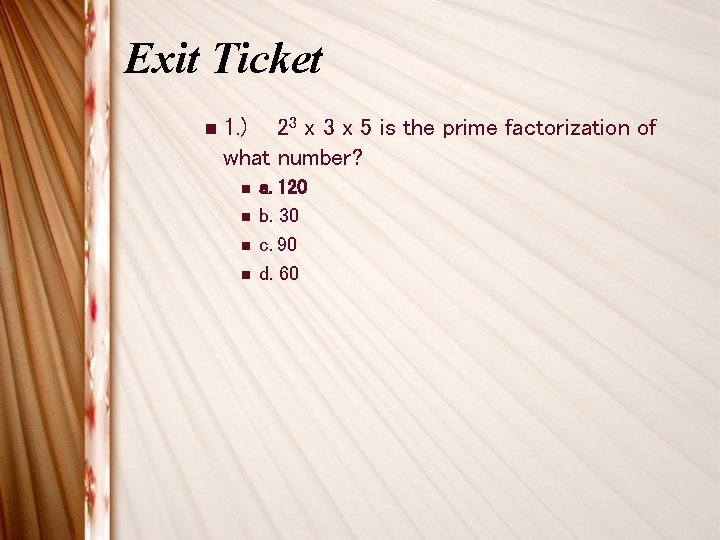Exit Ticket 23 x 5 is the prime factorization of what number? n 1. ) n n a. 120 b. 30 c. 90 d. 60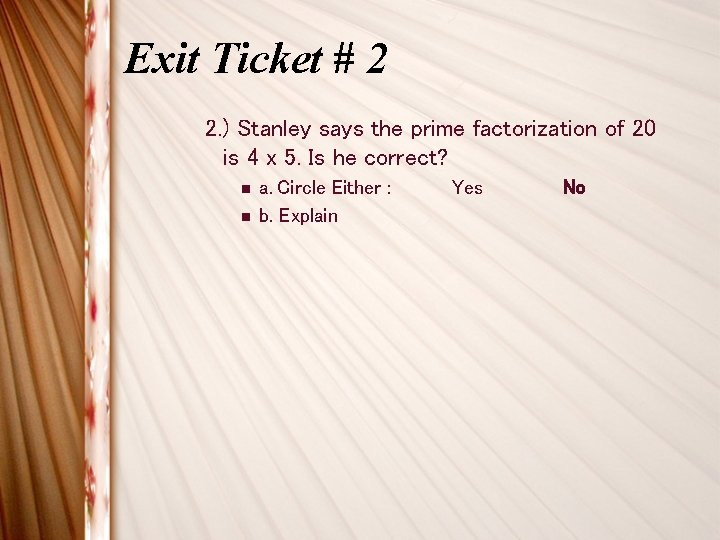Exit Ticket # 2 2. ) Stanley says the prime factorization of 20 is 4 x 5. Is he correct? n n a. Circle Either : b. Explain Yes NoPrime Factorization n 3. ) What is the prime factorization of 150?